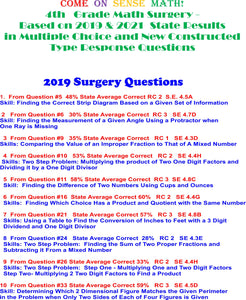# Digital Come on Sense 4th Grade Math Surgery Based on Most Difficult Questions From 2019 & 2021 Tests in Multiple Choice AND Constructed Type Response Formats!

• \$15.00
• Save \$5

COME ON SENSE MATH  USING THE FIVE SENSES

\$15 until Test Day

4th Grade Math Surgery/Practice Test Based on Questions                                      Modeled After Most Difficult Questions/TEKS from 2019 & 2021 Tests

NOW IN BOTH OPEN ENDED CONSTRUCTED TYPE RESPONSE AND MULTIPLE CHOICE FORMATS FOR BOTH YEARS!!!!!

This product will be sent to you as a test that has all 24 questions modeled for you one question at a time in short easy access You Tube videos that you can show your students based on whatever specific TEKS or skills you are going over at different times of the year.  The complete pdf of the practice test itself will be sent to you digitally by email once you complete your purchase at checkout in the store.  Includes: Strategies for each question modeled one at a time on You tube/Facebook, Full Color Math Posters, and Graphic Organizers for Place Value, Problem Solving Checklist, and Key Words for Operations Chart

2019 QUESTIIONS IN CONSTRUCTED TYPE AND MULTIPLE CHOICE FORMATS

1. Similar to Question #5 48% State Average Correct

Strip Diagrams with Two Steps of Division

RC 2 S.E. 4.5A

2 Similar to Question #6 30% State Average Correct

Using a protractor to find the measurement of an angle with two rays NOT going through zero

RC 3 SE 4.7D

3 Similar to Question #9 35% State Average Correct                                                     Comparing improper and mixed fractions using grams

RC 1 SE 4.3D

4 Similar to  Question #10 53% State Average Correct

Two Step Problem: Finding the remainder after multiplying and dividing one and two digit numbers

RC 2 SE 4.4H

5 Similar to Question #11 58% State Average Correct                                                    Finding the total number of cups and ounces of sugar left unused after baking a cake

RC 3 SE 4.8C

6 Similar to Question #16 State Average Correct 60%                                                 Finding out which pair of expressions have a quotient & product of ABOUT  9

RC 2 SE 4.4G

7 Similar to Question #21 State Average Correct 57%                                                   Converting inches of a poster to feet

RC 3 SE 4.8B

8 Similar to Question #24 State Average Correct 28%

Two Step Problem: Using Fractions and time to combine one set of numbers and the difference of another number

RC 2 SE 4.3E

9 Similar to Question #26 State Average Correct 33%

Open ended question needing two steps of two and three digit multiplication

RC 2 SE 4.4H

10 Similar to Question #33 State Average Correct 59%                                                   Comparing the perimeters of four rectangles with only two sides shown on each figure

RC 3 SE 4.5D

2021 Constructed Respone & Multiple Choice Formatted Questions

11 From Question #8 State Average Correct 39% RC 1 S.E. 4.2G Readiness
Skills: Equations that Show Equivalent Decimals and Fractions

12 From Question #9 State Average Correct 52% RC 2 S.E. 4.5A Readiness
Skill: Sets of Equations that Find a Missing Value

13 From Question #12 State Average Correct 45% RC 3 S.E. 4.7D Readiness
Skill: Using a Protractor to Construct a Ray to Find a Specific Angle

14 From Question #13 State Average Correct 51% RC 4 S.E. 4.9A Readiness
Skills: Using Dot Plots to Match a Lists of Lengths, Using the Ruler to Find
a Missing Length

15 From Question #14 State Average Correct 33% RC 3 S.E. 4.5D Readiness
Skill: Finding the Area of a Square When Only One Side is Given

16 From Question #15 State Average Correct 18% RC 2 S.E. 4.4H Readiness
Skills: Multiplication of Two 2 Digit Factor and Dividing a One Digit Divisor

17 From Question #16 State Average Correct 35% RC 4 S.E. 4.10B Supporting
Skills: Finding the Difference Between the Cost of Items and Their Selling
Prices to Determine the Amount of Profit

18 From Question #17 State Average Correct 48% RC 3 S.E. 4.6C Supporting
Skills: Attributes of a Given Type of Triangle

19 From Question #20 State Average Correct 51% RC 1 S.E. 4.2E Supporting
Skill: Using a Model to Find the Value of the Shaded/Unshaded Portion of a
Decimal Less Than One in Word Form

20 From Question #24 State Average Correct 43% RC 1 S.E. 4.2B Readiness
Skill: Using Three Attributes of a Number and Place Value

21 From Question #25 State Average Correct 50% RC 2 S.E. 4.3E Readiness
Skill: Finding a Missing Fraction Using Given Information and a Model

22 From Question #27 State Average Correct 53% RC 2 S.E. 4.4H Readiness
Skills: Two Step Problems: Multiplication of One and Two Digit Factors Divided
by a One Digit Divisor

23 From Question #29 State Average Correct 43% RC 3 S.E. 4.8C Readiness
Skill: Finding the Total Weight of Two Given Objects in Pounds & Ounces
When the Total Ounces Add to More than 1 Pound/16 Ounces

24 From Question #31 State Average Correct 52% RC 3 S.E. 4.7C Readiness
Skill: Finding an Angle with a Given Measurement Using 4 Protractors